# Parallel displacement(2)

(diff) ← Older revision | Latest revision (diff) | Newer revision → (diff)

An isomorphism of fibres over the end-pointsand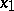of a piecewise-smooth curve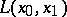in the baseof a smooth fibre spacedefined by some connection given in; in particular, a linear isomorphism between the tangent spaces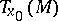anddefined along a curveof some affine connection given on. The development of the concept of a parallel displacement began with the ordinary parallelism on the Euclidean plane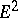, for which F. Minding (1837) indicated a way of generalizing it to the case of a surfaceinby means of the development of a curve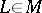onto the plane, a notion he introduced. This served as the starting point for T. Levi-Civita , who, by forming analytically a parallel displacement of the tangent vector to a surface, discovered that it depends only on the metric of the surface and on this basis generalized it at once to the case of an-dimensional Riemannian space (see Levi-Civita connection). H. Weyl  placed the concept of parallel displacement of a tangent vector at the base of the definition of an affine connection on a smooth manifold. Further generalizations of the concept are linked with the development of a general theory of connections.

Suppose that on a smooth manifoldan affine connection is given by means of the matrix of local connection forms:One says that a vector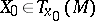is obtained by parallel displacement from a vector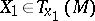along a smooth curve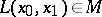if onthere is a smooth vector fieldjoining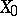and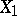and such that. Hereis the field of the tangent vector ofand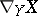is the covariant derivative ofrelative to, which is defined by the formula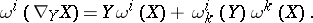Thus, the coordinates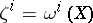ofmust satisfy alongthe system of differential equationsFrom the linearity of this system it follows that a parallel displacement alongdetermines a certain isomorphism betweenand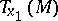. A parallel displacement along a piecewise-smooth curve is defined as the composition of the parallel displacements along its smooth pieces.

The automorphisms of the space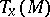defined by parallel displacements along closed piecewise-smooth curves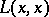form the linear holonomy group; hereand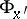are always conjugate to each other. If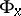is discrete, that is, if its component of the identity is a singleton, then one talks of an affine connection with a (local) absolute parallelism of vectors, or of a (locally) flat connection. Then the parallel displacement for anyanddoes not depend on the choice offrom one homotopy class; for this it is necessary and sufficient that the curvature tensor of the connection vanishes.

On the basis of the parallel displacement of a vector one defines the parallel displacement of a covector and, more generally, of a tensor. One says that the field of a covectoronaccomplishes a parallel displacement if for any vector fieldonaccomplishing the parallel displacement the function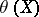is constant along. More generally, one says that a tensor fieldof type, say, accomplishes a parallel displacement alongif for any,andaccomplishing a parallel displacement the function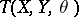is constant along. For this it is necessary and sufficient that the components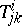satisfy alongthe system of differential equations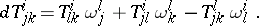After E. Cartan introduced in the 1920's  a space of projective or conformal connection and the general concept of a connection on a manifold, the notion of parallel displacement obtained a more general content. In its most general meaning it is considered nowadays as the analysis of connections in principal fibre spaces or fibre spaces associated to them. There is a way of defining the very concept of a connection by means of that of parallel displacement, which is then defined axiomatically. However, a connection can be given by a horizontal distribution or some other equivalent manner, for example, a connection form. Then for every curvein the baseits horizontal liftings are defined as integral curves of the horizontal distribution over. A parallel displacement is then the name for a mapping that puts the end-points of these liftings in the fibre overinto correspondence with their other end-points in the fibre over. The concepts of the holonomy group and of a (locally) flat connection are defined similarly; the latter are also characterized by the vanishing of the curvature form.

How to Cite This Entry:
Parallel displacement(2). Encyclopedia of Mathematics. URL: http://encyclopediaofmath.org/index.php?title=Parallel_displacement(2)&oldid=16862
This article was adapted from an original article by Ãœ. Lumiste (originator), which appeared in Encyclopedia of Mathematics - ISBN 1402006098. See original article